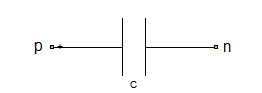# capacitor

Capacitor object

## Description

Use the `capacitor` class to create a capacitor object that you can add to an existing circuit.## Creation

### Syntax

``cobj = capacitor(cvalue)``
``cobj = capacitor(cvalue,cname)``

### Description

example

````cobj = capacitor(cvalue)` creates a capacitor object, `cobj`, with a capacitance of `cvalue` and default name, `C`.`cvalue` must be a real scalar.```

example

````cobj = capacitor(cvalue,cname)` creates a capacitor object, `cobj`, with a capacitance of `cvalue` and name `cname`. `cname` must be a character vector.```

## Properties

expand all

Capacitance value specified as a real scalar in farads.

Example: `1e-12`

Example: `cobj.Capacitance = 1e-12`

Name of capacitor object, specified as a character vector. Two elements in the same circuit cannot have the same name.

Example: `'cap'`

Example: `cobj.Name = 'cap'`

Names of the terminals of capacitor object, specified as a cell vector. These names are always `p` and `n`.

Example: `{'p' 'n'}`

Example: `cobj.Terminals = {'p' 'n'}`

Full path of the circuit to which the capacitor object belongs, specified as character vector. This path appears only after the capacitor is added to the circuit.

Note

"ParentPath" is only displayed after the capacitor has been added

into a circuit.

Circuit nodes in the parent nodes connect to capacitor terminals, specified as a vector of integers. This property appears only after the capacitor is added to a circuit.

Example: `[1 2]`

Example: `lobj.ParentNodes = [1 2]`

Note

"ParentNodes" are only displayed after the capacitor has been added

into a circuit.

## Object Functions

 `clone` Create copy of existing circuit element or circuit object

## Examples

collapse all

Create a capacitor of capacitance 2 microfarad and display its properties.

```hC1 = capacitor(2e-6); disp(hC1)```
``` capacitor: Capacitor element Capacitance: 2.0000e-06 Name: 'C' Terminals: {'p' 'n'} ```

Create a capacitor and extract S-parameters of the capacitor.

```hC = capacitor(2e-6,'C2uf'); hckt = circuit('example2'); add(hckt,[1 2],hC) setports(hckt, [1 0],[2 0]) freq = linspace(1e3,2e3,100); S = sparameters(hckt,freq); disp(S)```
``` sparameters: S-parameters object NumPorts: 2 Frequencies: [100x1 double] Parameters: [2x2x100 double] Impedance: 50 rfparam(obj,i,j) returns S-parameter Sij ```

Add capacitor to a circuit, display the parent path and parent nodes.

```hC3 = capacitor(3e-6,'C3uf'); hckt3 = circuit('example3'); add(hckt3,[1 2],hC3) setports(hckt3, [1 0],[2 0]) disp(hC3)```
``` capacitor: Capacitor element Capacitance: 3.0000e-06 Name: 'C3uf' Terminals: {'p' 'n'} ParentNodes: [1 2] ParentPath: 'example3' ```

## Version History

Introduced in R2013b

expand all